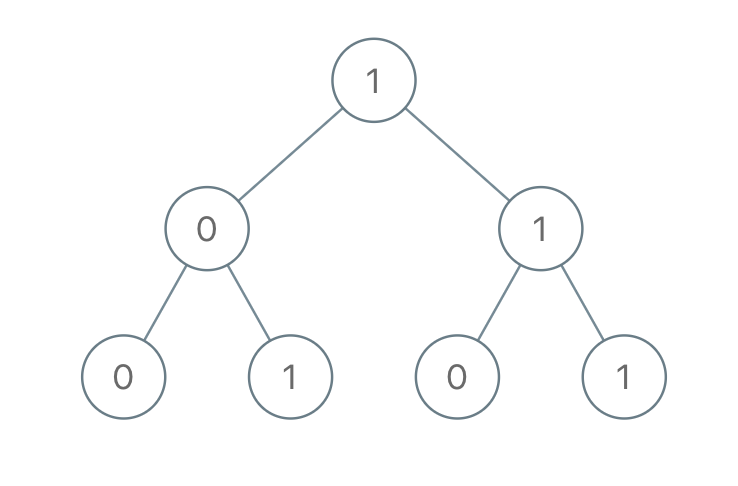### LeetCode - Tree -Sum of Root To Leaf Binary Numbers

Given a binary tree, each node has value `0` or `1`.  Each root-to-leaf path represents a binary number starting with the most significant bit.  For example, if the path is `0 -> 1 -> 1 -> 0 -> 1`, then this could represent `01101` in binary, which is `13`.

For all leaves in the tree, consider the numbers represented by the path from the root to that leaf.

Return the sum of these numbers.

Example 1:```Input: [1,0,1,0,1,0,1]
Output: 22
Explanation: (100) + (101) + (110) + (111) = 4 + 5 + 6 + 7 = 22```

```/**  * Definition for a binary tree node.  * public class TreeNode {  *     int val;  *     TreeNode left;  *     TreeNode right;  *     TreeNode(int x) { val = x; }  * }  */ class Solution {     List<String> numList = new ArrayList<String>();     public int sumRootToLeaf(TreeNode root) {         TreeNode node = root;         String num = "";                 int total = 0;                  getNum(num, node);         //System.out.println(numList);                  for(String n : numList){             int s = Integer.parseInt(n,2);             total = total+s;         }                  return total;     }          public void getNum(String rootStr, TreeNode node){         if(node.left !=null) {             getNum(rootStr+node.val,node.left);         }         if(node.right != null){             getNum(rootStr+node.val,node.right);         }         if(node.right == null && node.left == null){             rootStr = rootStr+node.val;             numList.add(rootStr);         }     } }```